# Cube + diagonal - math problems

#### Number of problems found: 36

• Sphere vs cubeHow many % of the surface of a sphere of radius 12 cm is the surface of a cube inscribed in this sphere?
• Cube in sphereThe cube is inscribed in a sphere with a radius r = 6 cm. What percentage is the volume of the cube from the volume of the ball?
• Cube diagonalsThe cube has a wall area of 81 cm square. Calculate the length of its edge, wall, and body diagonal.
• Wall diagonalCalculate the length of wall diagonal of the cube whose surface is 384 cm square.
• CubeCalculate the surface of the cube ABCDA'B'C'D' if the area of rectangle ACC'A' = 344 mm2.
• Body diagonalCalculate the volume and surface of the cube if the diagonal body measures ten dm.
• Body diagonalFind the cube surface if its body diagonal has a size of 6 cm.
• Cube diagonalsCalculate the length of the side and the diagonals of the cube with a volume of 27 cm3.
• Inscribed circle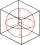A circle is inscribed at the bottom wall of the cube with an edge (a = 1). What is the radius of the spherical surface that contains this circle and one of the vertex of the top cube base?
• Calculate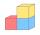Calculate the length of the wall diagonal of the cube with an edge 5 cm long.
• Cube cut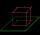The cube ABCDA'B'C'D ' has an edge of 12cm. Calculate the area of diagonal cut B DD'B '.
• Cube in sphereThe sphere is an inscribed cube with an edge of 8 cm. Find the sphere's radius.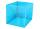I have homework. The cube has an edge 7 cm long and I must find wall and body diagonal.
• Body diagonalCalculate the length of the body diagonal of the 6cm cube.
• Cube diagonalDetermine the length of the cube diagonal with edge 37 mm.
• Cube - wall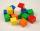V kocke ABCDEFGH je |CF|=4 √ 2. Aký je povrch kocky?

Do you have an exciting math question or word problem that you can't solve? Ask a question or post a math problem, and we can try to solve it.

We will send a solution to your e-mail address. Solved examples are also published here. Please enter the e-mail correctly and check whether you don't have a full mailbox.

Cube Problems. Diagonal - math problems.